Function Repository Resource:

# PolynomialToBSplineCurve

Make a B-spline representation of a polynomial over an interval

Contributed by: Jan Mangaldan
 ResourceFunction["PolynomialToBSplineCurve"][p,{x,xmin,xmax}] generates a BSplineCurve representation of the polynomial p as a function of x from xmin to xmax.

## Details

ResourceFunction["PolynomialToBSplineCurve"] treats the variable x as local, effectively using Block.
ResourceFunction["PolynomialToBSplineCurve"] has attribute HoldAll and evaluates p only after assigning specific numerical values to x.
In some cases, it may be more efficient to use Evaluate to evaluate p symbolically before specific numerical values are assigned to x.

## Examples

### Basic Examples (1)

Use PolynomialToBSplineCurve to plot a polynomial:

 In:=Out=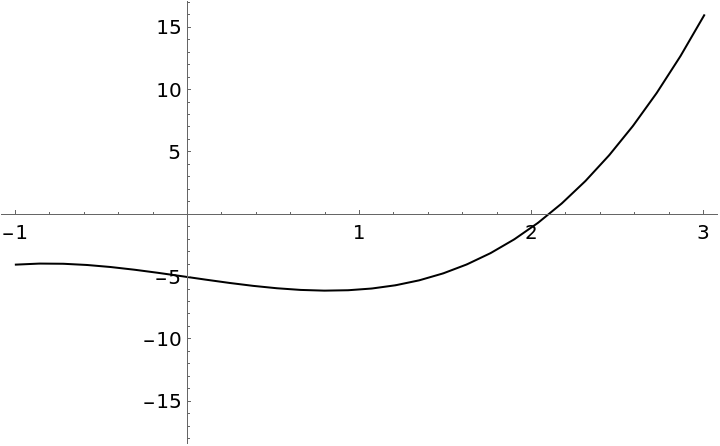### Scope (2)

Use a style similar to Plot:

 In:=Out=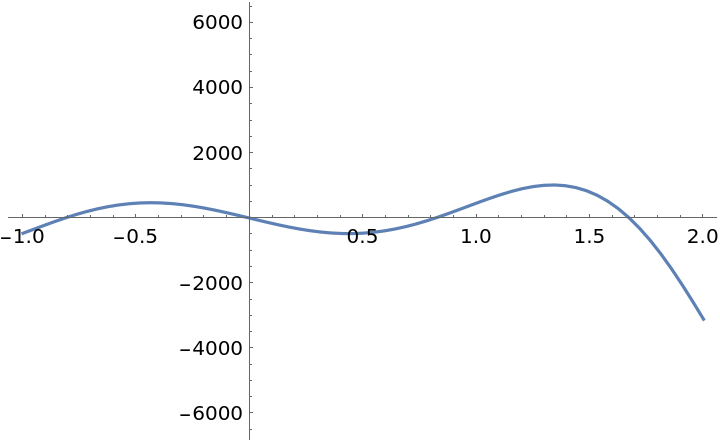Plot multiple polynomials:

 In:=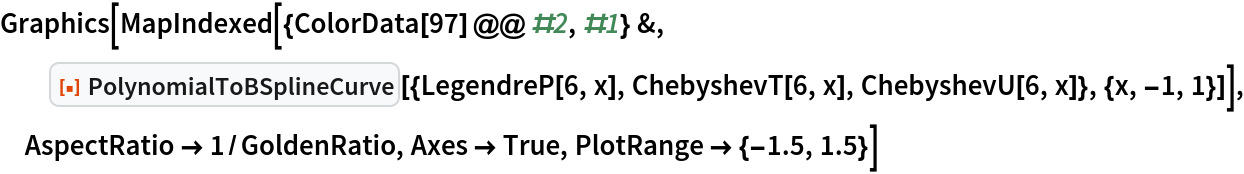Out=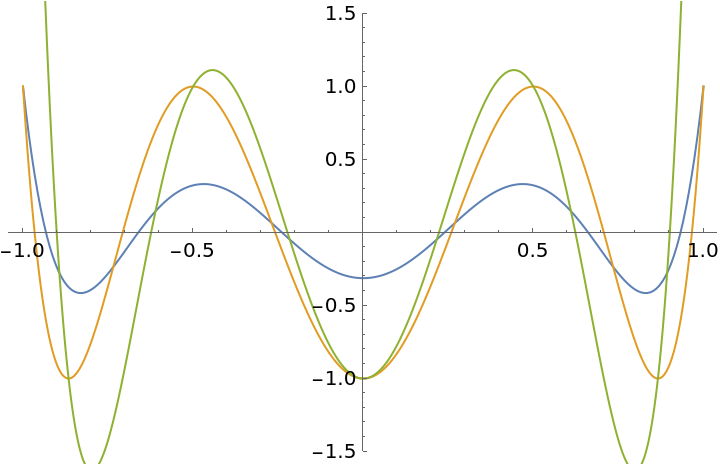### Properties and Relations (2)

PolynomialToBSplineCurve returns a BSplineCurve object:

 In:=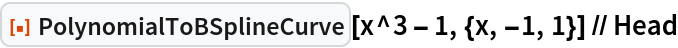Out=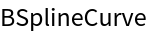Using PolynomialToBSplineCurve in Graphics usually gives results with a smaller ByteCount:

 In:=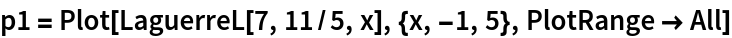Out=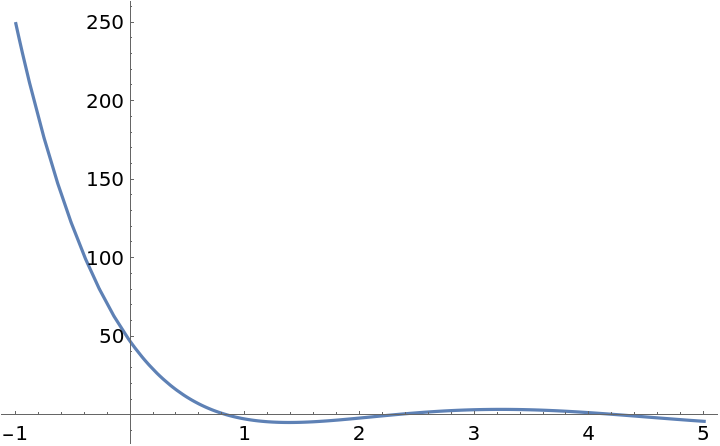In:=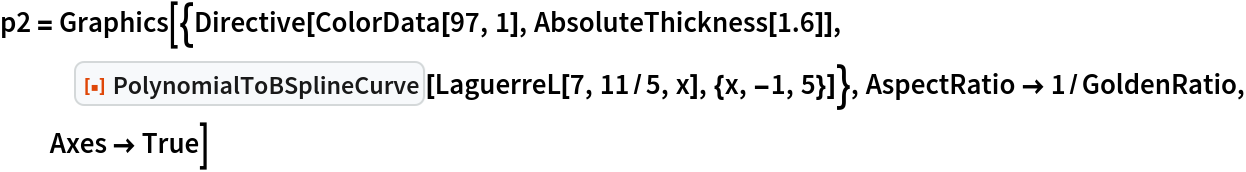Out=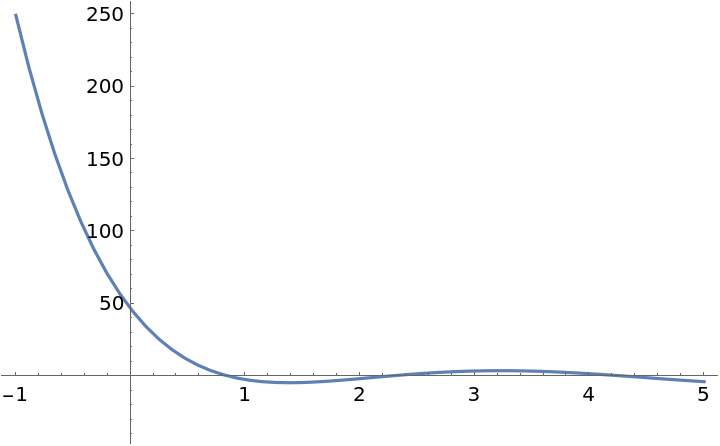In:=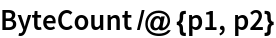Out=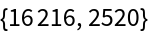## Version History

• 1.0.0 – 07 July 2021